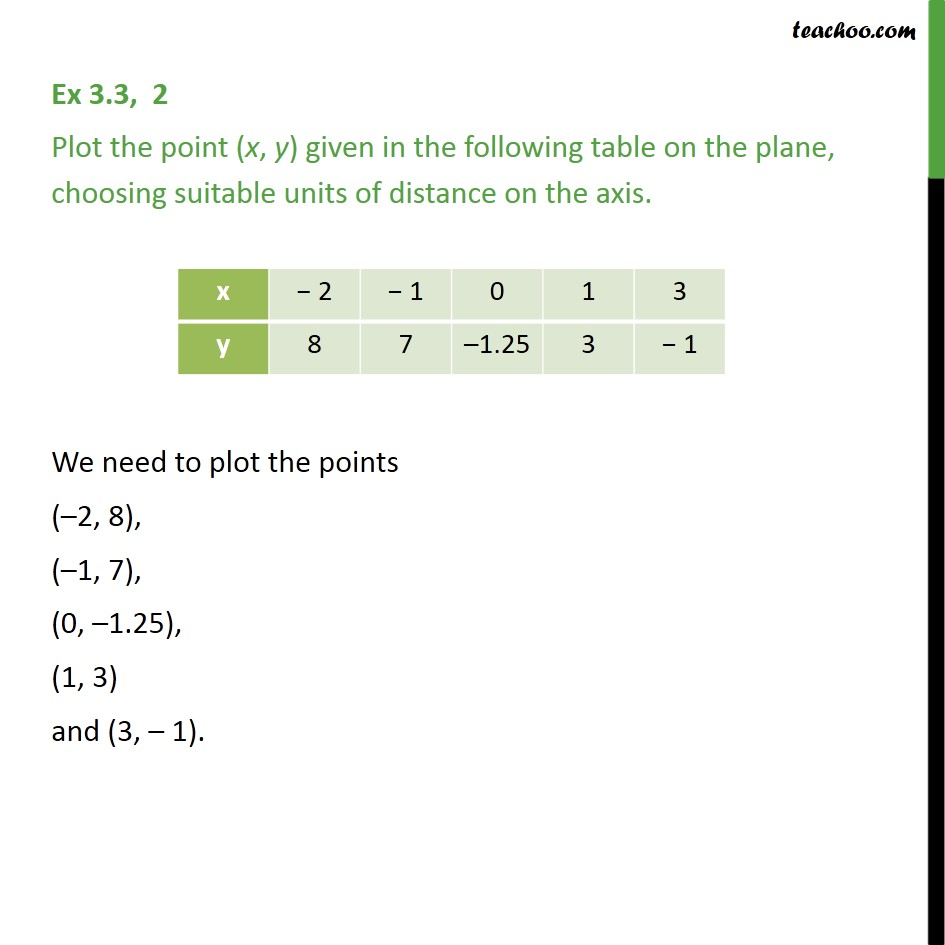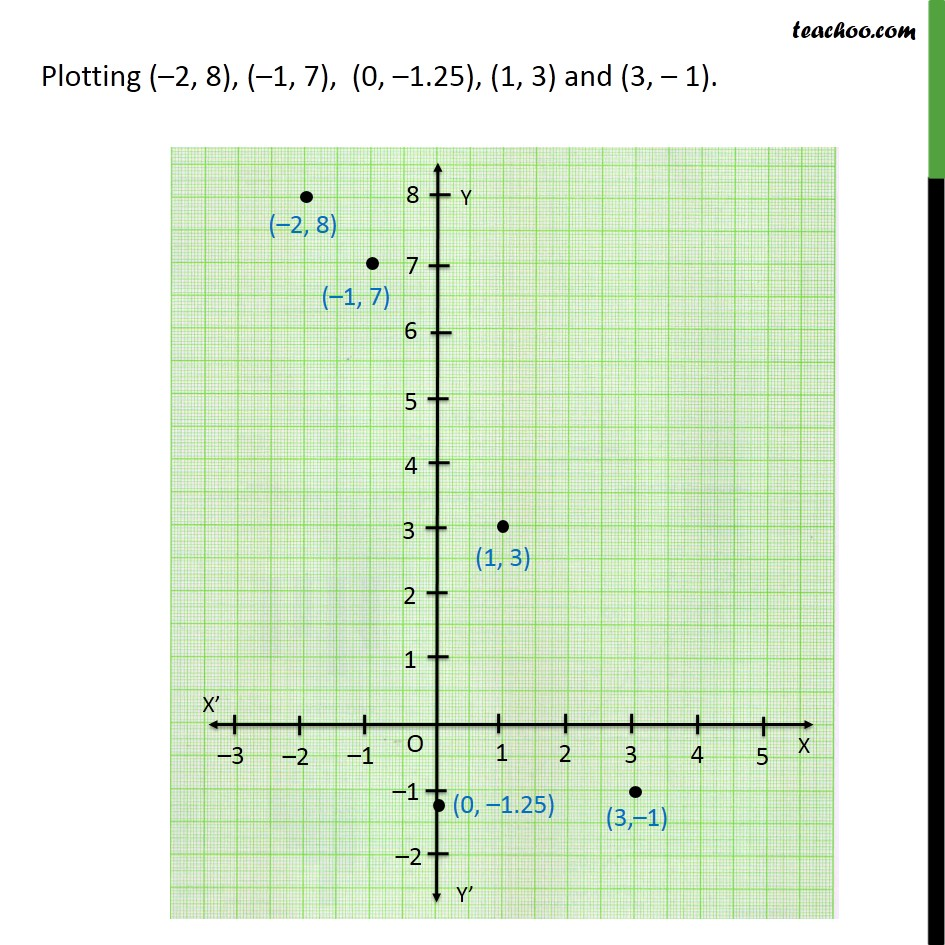1. Class 9
2. Important Questions for Exam - Class 9
3. Chapter 3 Class 9 Coordinate Geometry

Transcript

Ex 3.3, 2 Plot the point (x, y) given in the following table on the plane, choosing suitable units of distance on the axis. We need to plot the points ( 2, 8), ( 1, 7), (0, 1.25), (1, 3) and (3, 1). Plotting ( 2, 8), ( 1, 7), (0, 1.25), (1, 3) and (3, 1).

Chapter 3 Class 9 Coordinate Geometry

Class 9
Important Questions for Exam - Class 9